#Homogeneous Linear EquationsConsider the homogeneous second order linear equation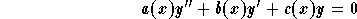or the explicit one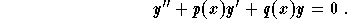Basic property:If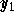and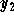are two solutions, then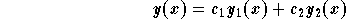is also a solution for any arbitrary constants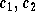.

The natural question to ask is whether any solution y is equal to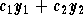for some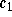and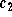.
The answer to this question uses the notion of linear independence of solutions.[Differential Equations] [First Order D.E.] [Second Order D.E.]
[Geometry] [Algebra] [Trigonometry ]
[Calculus] [Complex Variables] [Matrix Algebra]S.O.S MATHematics home page

Do you need more help? Please post your question on our S.O.S. Mathematics CyberBoard.Author: Mohamed Amine Khamsi